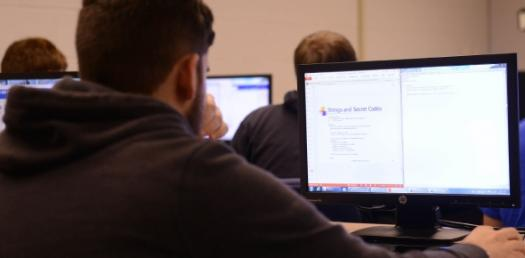# Computer Logic

9 Questions | Total Attempts: 153SettingsThis is a quiz about ligic gates for form 3 students taking Computer Studies 0 Level.

Related Topics
• 1.
What is this gate called?
• A.

NOT GATE

• B.

AND GATE

• C.

OR GATE

• 2.
How many inputs does this gate have?
• A.

1

• B.

2

• C.

3

• 3.
How many outputs does this gate have?
• A.

1

• B.

2

• C.

3

• 4.
What is the logic equivalent of True?
• A.

0

• B.

1

• C.

2

• 5.
What does a 0 represent in digital logic?
• A.

True

• B.

False

• C.

Unknown

• 6.
What is this gate called?
• A.

OR Gate

• B.

AND Gate

• C.

NOT Gate

• 7.
What is the function of this gate?
• A.

It produces the inverse of the input

• B.

It does not have any effect on the input

• C.

It changes the input randomly

• 8.
What is this gate called?
• A.

AND Gate

• B.

NOT Gate

• C.

OR Gate

• 9.
When I have more than one logic gate connected together, what do we call this?
• A.

Logic Circuit

• B.

Wired Circuit

• C.

Functional Circuit